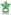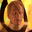Aamon Agrégat Poussiéreux• Male
• from Paris
• Member since Jul 26th 2017
Last Activity

This site uses cookies. By continuing to browse this site, you are agreeing to our Cookie Policy.

•Aamon - Aug 8th 2017, 3:48am

Orbital elements of the Sun:
N = 0.0
i = 0.0
w = 282.9404 + 4.70935E-5 * d
a = 1.000000 (AU)
e = 0.016709 - 1.151E-9 * d
M = 356.0470 + 0.9856002585 * d

Orbital elements of Mars:
N = 49.5574 + 2.11081E-5 * d
i = 1.8497 - 1.78E-8 * d
w = 286.5016 + 2.92961E-5 * d
a = 1.523688 (AU)
e = 0.093405 + 2.516E-9 * d
M = 18.6021 + 0.5240207766 * d

Orbital Element of our Home World (Earth)
E = M + e*(180/pi) * sin(M) * ( 1.0 + e * cos(M) )

for (int i = 0; i < 2; i++) {
xh = r * ( cos(N) * cos(v+w) - sin(N) * sin(v+w) * cos(i) )
yh = r * ( sin(N) * cos(v+w) + cos(N) * sin(v+w) * cos(i) )
zh = r * ( sin(v+w) * sin(i) )
CPPos = zh
}

Ainsi la magie prend forme, Le soleil, La Terre et Mars